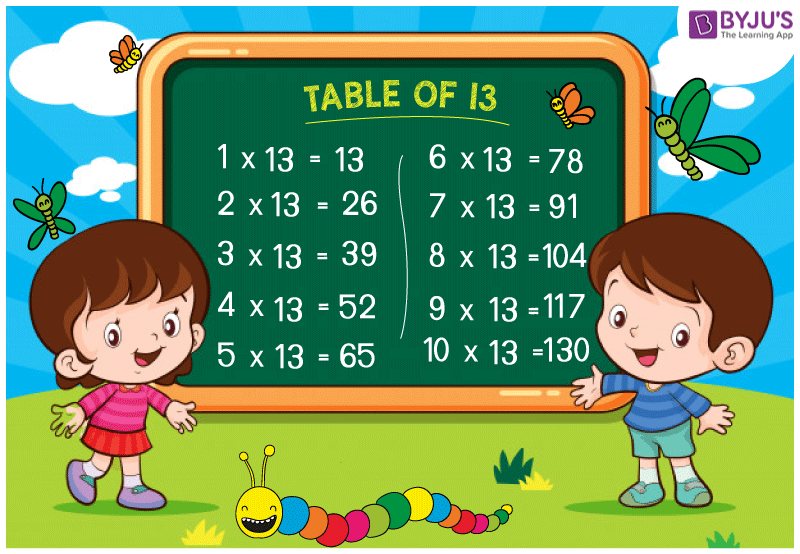# Table Of 13

Table of 13 is provided here so that students and candidates who are preparing for competitive exams, can have a look while solving questions. Table of 13 is also important for the students of class 9 onwards. It is also recommended to memorize the table until 20. Memorising the tables will reduce the time while multiplying the numbers in the exams to solve the problem.To help the students we have provided the 13 times table here.

Table of 13

 13 × 1 = 13 13 × 2 = 26 13 × 3 = 39 13 × 4 = 52 13 × 5 = 65 13 × 6 = 78 13 × 7 = 91 13 × 8 = 104 13 × 9 = 117 13 × 10 = 130 13 × 11 = 143 13 × 12 = 156 13 × 13 = 169 13 × 14 = 182 13 × 15 = 195 13 × 16 = 208 13 × 17 = 221 13 × 18 = 234 13 × 19 = 247 13 × 20 = 260

## Tables from 13 to 20

Tables from 13 to 20 are important to memorise to save time on calculations during exams. Once you know all the tables from 1 to 20, You will be able to make fast calculations. This will ultimately help you in saving time in calculating and gives you more time to solve the problem in exams.

Visit BYJU’S for latest CBSE and NCERT study materials. We also provide multiplication tables, class-wise notes for all the subjects.

Get More Maths Tables:-

Test your knowledge on Table Of 13